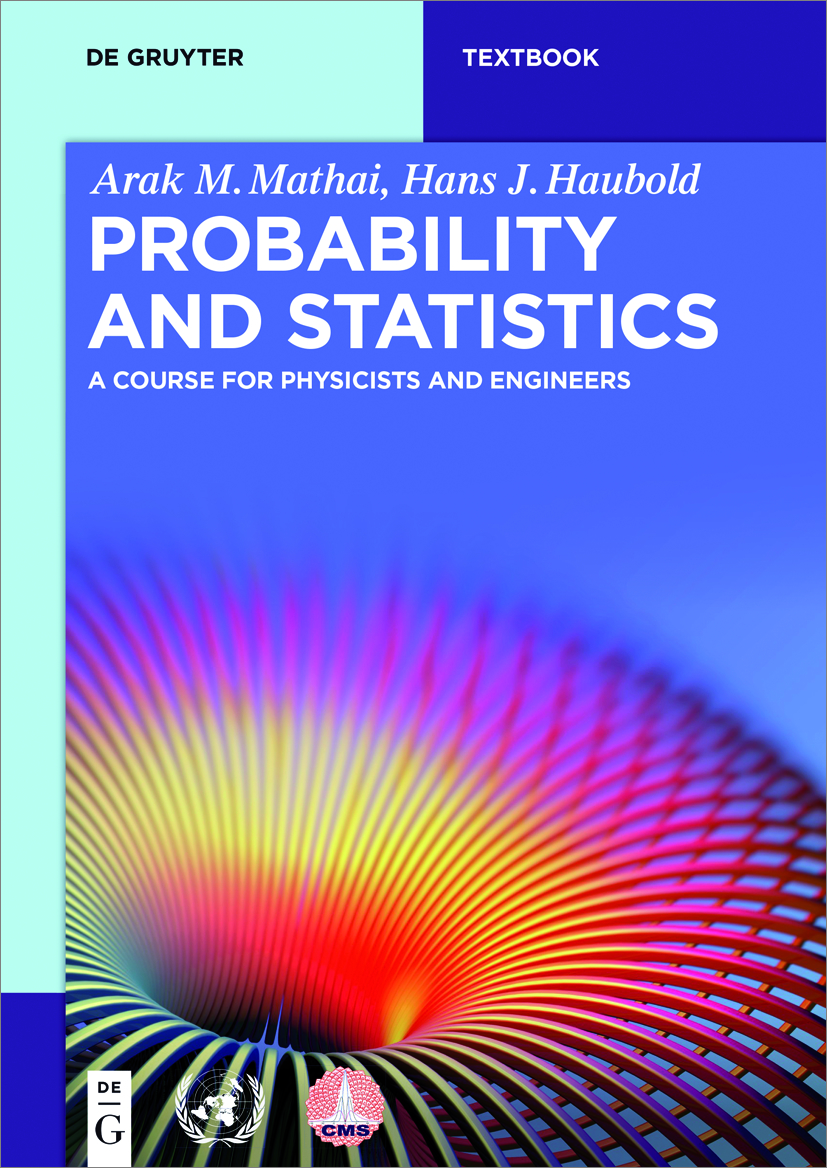News Release

# Probability and Statistics

A Course for Physicists and Engineers

De Gruyterimage: This book offers an introduction to concepts of probability theory, probability distributions relevant in the applied sciences, as well as basics of sampling distributions, estimation and hypothesis testing. As a companion for classes for engineers and scientists, the book also covers applied topics such as model building and experiment design. view more  Credit: De Gruyter

This book offers an introduction to concepts of probability theory, probability distributions relevant in the applied sciences, as well as basics of sampling distributions, estimation and hypothesis testing. As a companion for classes for engineers and scientists, the book also covers applied topics such as model building and experiment design.

Contents:

Random phenomena

Probability

Random variables

Expected values

Commonly used discrete distributions

Commonly used density functions

Joint distributions

Some multivariate distributions

Collection of random variables

Sampling distributions

Estimation

Interval estimation

Tests of statistical hypotheses

Model building and regression

Design of experiments and analysis of variance